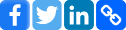Select Page

<script language=”JavaScript”>

// Parameters are rate, total number of periods, payment made each period and future value
function pv(rate, nper, pmt, fv) {
rate = parseFloat(rate);
nper = parseFloat(nper);
pmt = parseFloat(pmt);
fv = parseFloat(fv);
if ( nper == 0 ) {
alert(“Why do you want to test me with zeros?”);
return(0);
}
if ( rate == 0 ) { // Interest rate is 0
pv_value = -(fv + (pmt * nper));
} else {
x = Math.pow(1 + rate, -nper);
y = Math.pow(1 + rate, nper);
pv_value = – ( x * ( fv * rate – pmt + y * pmt )) / rate;
}
pv_value = pv_value.toFixed(2);
return (pv_value);
}

function fv(rate, nper, pmt, pv) {
rate = parseFloat(rate);
nper = parseFloat(nper);
pmt = parseFloat(pmt);
pv = parseFloat(pv);
if ( nper == 0 ) {
alert(“Why do you want to test me with zeros?”);
return(0);
}
if ( rate == 0 ) { // Interest rate is 0
fv_value = -(pv + (pmt * nper));
} else {
x = Math.pow(1 + rate, nper);
fv_value = – ( -pmt + x * pmt + rate * x * pv ) /rate;
}
fv_value = fv.toFixed(2);
return (fv_value);
}

function pmt(rate, payments, pv, fv, advancedpmts) {
var t1 = 1 + rate
var t2 = Math.pow(t1,payments)
var t3 = Math.pow(t1,(payments – advancedpmts))
return (pv – (fv/t2))/(((1-(1/(t3))) / rate) + advancedpmts);
}

</script>

Share this page: